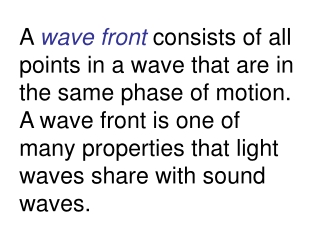DownloadDownload PresentationEx. 1 - What is the minimum mirror height necessary for a person to see their full image?

# Ex. 1 - What is the minimum mirror height necessary for a person to see their full image?

Download Presentation## Ex. 1 - What is the minimum mirror height necessary for a person to see their full image?

- - - - - - - - - - - - - - - - - - - - - - - - - - - E N D - - - - - - - - - - - - - - - - - - - - - - - - - - -
##### Presentation Transcript

1. A wave front consists of all points in a wave that are in the same phase of motion. A wave front is one of many properties that light waves share with sound waves.

2. A radial line pointing outward from a light source and perpendicular to the wave front is called a ray. Rays point in the direction of the velocity of the wave.

3. Waves whose wave fronts are flat surfaces are called plane waves. The rays for a plane wave are parallel to each other. A ray is a narrow beam of light.

4. The law of reflection states that the incident ray, the reflected ray, and the normal all lie in the same plane, and the angle of reflection θr equals the angle of incidence θi: θr = θi.

5. When light rays strike a smooth surface, reflected rays are parallel (as in a mirror), this is called specular reflection. Light is reflected in various directions when it strikes an irregular surface, this is called diffuse reflection.

6. Properties of the image in a plane mirror:1. upright (erect)2. same size3. same distance into mirror as object’s distance from mirror.

7. The rays that we see reflected from a plane mirror seem to emanate from a point behind the mirror. The rays cannot really originate there so the image is called a virtual image.

8. Curved mirrors can produce images from which the light rays actually emanate. These are called real images.

9. Ex. 1 - What is the minimum mirror height necessary for a person to see their full image?

10. Center of curvature, C, is the center of the original sphere.Vertex, V, is the center of the mirror.

11. Principle axis of the mirror is the line drawn through C and V.Secondary axis is any other line drawn through C to the mirror.Normal is a radius from the point of incidence of a light ray.

12. Focal Point F of the mirror is the point on the PA where light rays close to and parallel to the PA converge.For a curved mirror, the focal point is one-half the distance to the center of curvature, one-half the radius of the sphere f = R/2, if the curvature of the mirror is gradual.

13. Focal length, f, is the distance from the vertex to the principle focus.Rays that lie close to the principle axis are paraxial rays(paraxial rays lie close to the principle axis, but are not necessarily parallel to it), f = R/2 is only valid for paraxial rays.

14. Rays that are far from the principle axis do not converge to a single point after reflection. The resulting image is blurred; this is called spherical aberration. It can be minimized by using a mirror with a small curvature (small diameter compared to radius of curvature).

15. A parabolic mirror has no spherical aberration, even if it is a large mirror.

16. Convex mirrors also have focal points with focal lengths that are one-half the radius of curvature. We assign the focal length of convex mirror a negative value: f = -R/2.

17. It is convenient to produce three rays that leave the same point on an object:Ray 1 is parallel to the principle axis and reflects through the focal point,Ray 2 passes through the focal point and reflects parallel to the principle axis,Ray 3 travels through the center of curvature and thus reflects back on itself.

18. Images formed by concave mirror:Case #1 Object at an infinite distance.

19. Case #3 Object at center of curvature

20. Case #5 Object at principle focus

21. Case #6 Object between principle focus and mirror

22. Convex mirrors

23. Mirror equations:1/f = 1/do + 1/di hi/ho = di/do

24. 1. The numerical value of the focal length f of a concave mirror is positive.2. The numerical value of the focal length f of a convex mirror is negative.

25. 3. The numerical value of the real object distance do is positive.4. The numerical value of the real image distance di is positive.5. The numerical value of the virtual image distance di is negative.

26. Ex. 3 - A 2.0-cm-high object is placed 7.10 cm from a concave mirror whose radius of curvature is 10.20 cm. Find (a) the location of the image and (b) its size.

27. Ex. 4 - An object is placed 6.00 cm in front of a concave mirror that has a 10.0-cm focal length. (a) Determine the location of the image. (b) If the object is 1.2 cm high, find the image height.

28. Ex. 5 - A convex mirror is used to reflect light from an object placed 66 cm in front of the mirror. The focal length of the mirror is f = -46 cm. Find (a) the location of the image and (b) the magnification.

29. Ex. 6 - An object is placed 9.00 cm in front of a mirror. The image is 3.00 cm closer to the mirror when the mirror is convex than when it is planar. Find the focal length of the convex mirror.